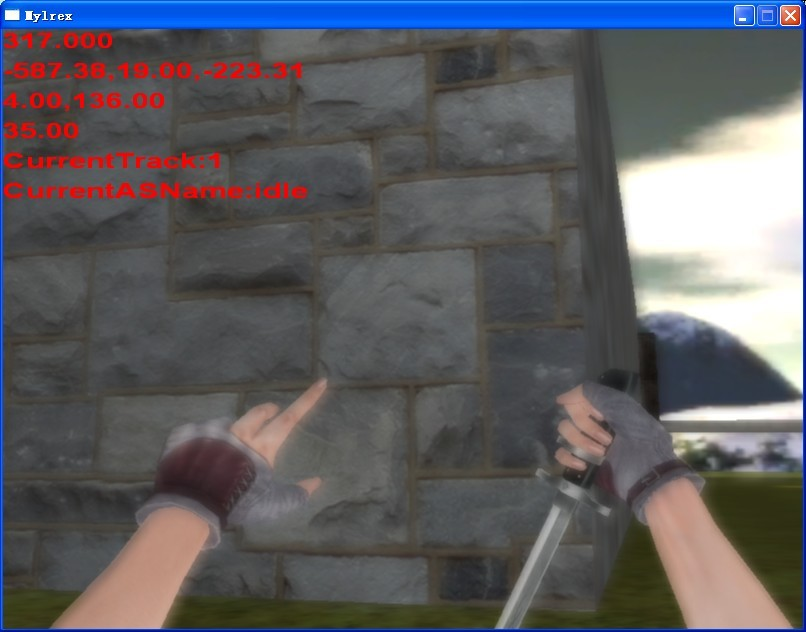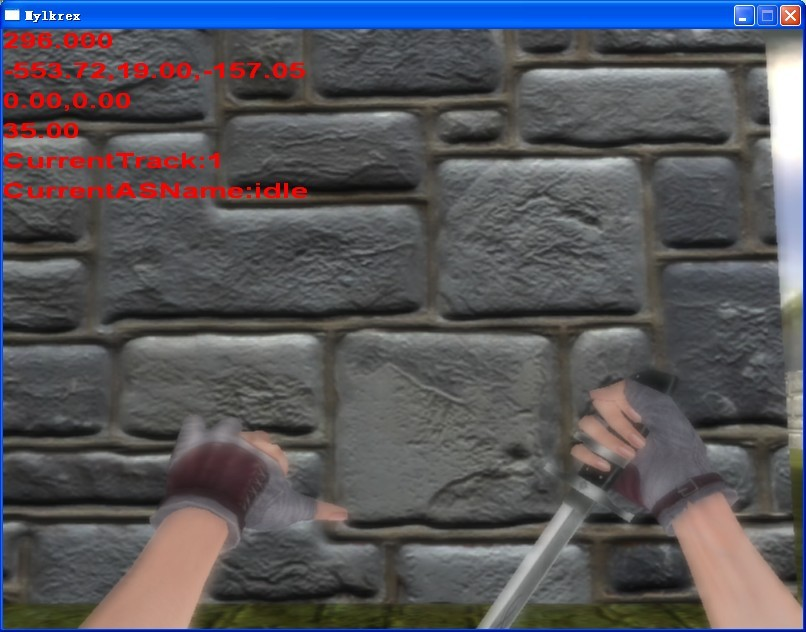2016/07/23 23:18

# 对于法线贴图的深入研究

## 正文

`法线贴图`技术中，我们就是通过把墙面的每个像素的法线存储在一张纹理中，渲染的时候根据每个像素的法线确定他们的阴暗程度，而这张`法线贴图`是可以用`photoshop`软件从一张墙的纹理生成对应的`法线贴图的`。到此，熟悉`法线贴图`的朋友会对以上内容很熟悉的。

`正切空间`中，三个基向量分别叫做`T``B``N``T`代表`Tangent`,`B`代表`Binormal`,`N`代表`Normal`。在`Texture Space``纹理空间`中）, 两个二维基向量分别叫做`U``V``T`就可以映射到纹理空间中的`U``B`就可以映射到`纹理空间`中的`V`。下面我们来推导一下三个向量的计算公式：

``````   pi = ui.T + vi.B
``````

``````   p1 = u1.T + v1.B
p2 = u2.T + v2.B
p3 = u3.T + v3.B
``````

``````   p2 - p1 = (u2 - u1).T + (v2 - v1).B
p3 - p1 = (u3 - u1).T + (v3 - v1).B
``````

``````       (v3 - v1).(p2 - p1) - (v2 - v1).(p3 - p1)
T = -----------------------------------------
(u2 - u1).(v3 - v1) - (v2 - v1).(u3 - u1)

(u3 - u1).(p2 - p1) - (u2 - u1).(p3 - p1)
B = -----------------------------------------
(v2 - v1).(u3 - u1) - (u2 - u1).(v3 - v1)
``````

`N`轴可以由两轴叉乘得到：

``````                        N = cross(T, B)
``````

``````                        |Tx Bx Nx|
|Ty By Ny|
|Tz Bz Nz|
``````

`正切空间``世界空间`转换：

``````   VWorld = TBN·VTangent = VTangentT·TBNT
``````

`世界空间``正切空间`转换：

``````   VTangent = TBN-1·VWorld = VWorldT·TBN-T
``````

``````  float3 normal = tex2D(NormapTex,inTxr) * 2 - 1;
float3 TBNLightPos = mul(lightPos,TBN_matrix);
float  DiffuseAttn =  clamp(0, 1,dot(normal, TBNLightPos ));
``````

`````` float3 normal = tex2D(NormapTex,inTxr) * 2 - 1;
float DiffuseAttn =  clamp(0, 1,dot(normal, float3(1,-1,1)));
``````0
0 收藏

### 作者的其它热门文章0 评论
0 收藏
0